Courses

Test: Polynomials (Hard)

20 Questions MCQ Test | Test: Polynomials (Hard)

Description
Attempt Test: Polynomials (Hard) | 20 questions in 20 minutes | Mock test for Class 10 preparation | Free important questions MCQ to study for Class 10 Exam | Download free PDF with solutions
QUESTION: 1

What must be subtracted from the polynomial 8x4 + 14x3 + x2 + 7x + (8 so that the resulting polynomial is exactly divisible by 4x2 - 3x + 2?

Solution: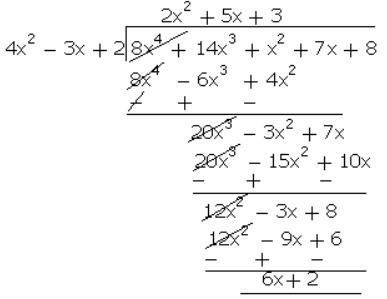Thus, when 6x + 2 is subtracted from the given polynomial 8x4 + 14x3 + x2 + 7x + 8, then it will be divisible by 4x2 - 3x + 2.

QUESTION: 2

Find the smallest solution in positive integers of x2 - 14y2 = 1

Solution: x2 − 1 = 14y2

Now checking by putting y = 1, 2, 3... until we get solution

y = 1; => x2 =15 not possible

y = 2; => x2 = 57 not possible

y = 3; => x2 = 127 not possible

y = 4; => x2 = 225 => x = 15

Hence, the smallest solution is (x, y) = (15, 4).

QUESTION: 3

Obtain all other zeroes of 3x4 + 6x3 - 2x2 - 10x - 5, if two of its zeros are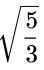and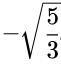.

Solution: Since two zeroes areand, x =, x =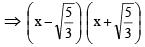= x2 - 5 / 3 or 3x2 – 5 is a factor of the given polynomial.

Now, we apply the division algorithm to the given polynomial and 3x2 – 5.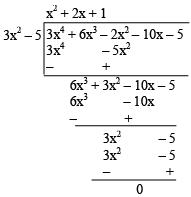So, 3x4 + 6x3 – 2x2 – 10x – 5 = (3x2 – 5) (x2 + 2x + 1) + 0

Quotient = x2 + 2x + 1 = (x + 1)2

Zeroes of (x + 1)2 are –1, –1.

Hence, all its zeroes are,, -1, -1

QUESTION: 4

The zeroes of the quadratic polynomial 4x2 + 87x + 125 are

Solution: 4x2 + 87x + 125 = 0

a = 4, b = 87, and c = 125

x= −b ± b2 − 4ac−−−−−−−√2a

x=−87 ± 872 − 4(4)(125)−−−−−−−−−−−−√2(4)

x= −87 ± 7569 − 2000−−−−−−−−−−√8

x= −87 ± 5569−−−−√8

The discriminant b2 − 4ac > 0

so, there are two real roots.

x = −87 ± 5569−−−−√8

x = −878 ± 5569−−−−√8

Simplify fractions and/or signs:

x = −878 ± 5569−−−−√8

which becomes x = −1.54678

x = −20.2032

QUESTION: 5

Zeroes of the quadratic polynomial x2 + 3x -(a + 2)(a - 1) is

Solution: The given polynomial is: P(x) = x2 + 3x - (a + 2)(a - 1)

Zeros of the polynomial obtained by taking P(x) = 0

x2 + 3x - (a + 2)(a - 1) = 0

x2 + (a + 2)x - (a - 1)x - (a + 2)(a - 1) = 0

x[x + a + 2] - (a - 1)[x + a + 2] = 0

[x + a + 2][x - (a - 1)] = 0

x = -(a + 2) or x = a - 1

Hence, the zeros of the polynomial are (a + 2) and (a - 1).

QUESTION: 6

The degree of ___ polynomials are not defined.

Solution:
• The value zero" 0" can be considered as a (constant) polynomial, called the zero polynomial.

• Like any other constant value, It has no nonzero terms.

• There we can say that it has no degree either.

• However, its degree is undefined.

Thus a zero is a polynomial of degree zero.

QUESTION: 7

A linear polynomial has one and only one ___.

Solution: Number of zeroes of a n - degree polynomial = n.

First, a linear polynomial is in the form of ax + b, a ≠ 0, a, b ∈ R

Degree of the polynomial = highest degree of the terms

So, here the highest degree is 1.

Hence, Linear polynomial has only one zero.

QUESTION: 8

A polynomial of degree n has at most ____ zeroes.

Solution: A polynomial of n degree can have n zeros.

For example: A quadratic equation ax2 + bx + c = 0 can have 2 zeros, as the highest power of x is 2 or as the degree is 2. ax3 + bx2 + cx + d = 0, a cubic equation can have 3 zeros, as the highest power of x is 3 or as the degree is 3.

QUESTION: 9

A polynomial having ____the term is called binomial.

Solution: In algebra, a binomial is a polynomial that is the sum of two terms, each of which is a monomial.
QUESTION: 10

The value of the polynomial f(x) = 4x2 - 7 at x = 2 is

Solution:

Given that x = 2

Substitute x in 4x2- 7

4(22) - 7

4(4) - 7

16 - 7 = 9

QUESTION: 11

If one zero of 3x - 8x + 2k + 1 is Severn times the other, then k is____.

Solution: 3x2 - 8x + 2k + 1

a = 3, b = -8 ,c = 2k + 1

suppose the zeroes of the equation are α and β. By the given condition α = 7β

As we know , α + β = -b / a

7β + β = -(-8) / 3

8β = 8 / 3

b = 8 / 3 × 1 / 8 = 1 / 3

αβ = c /a

7β × β = 2k + 1 / 3

7 × 1 / 3 × 1 / 3 = 2k + 1 / 3

7 / 9 = 2k + 1 / 3

21 / 9 = 2k + 1

21 / 9 - 1 = 2k

12 / 9 = 2k

4 / 3 = 2k

4 / 6 = k

2 / 3 = k

QUESTION: 12

The graph of a quadratic polynomial p(x) = 5x2 + 3x + 2 is an upward parabola.

Solution: There is an easy way to tell whether the graph of a quadratic function opens upward or downward: if the leading coefficient is greater than zero, the parabola opens upward, and if the leading coefficient is less than zero, the parabola opens downward.
QUESTION: 13

The graph of a quadratic polynomial q(x) = - 3x2 + 2 is an upward parabola.

Solution: There is an easy way to tell whether the graph of a quadratic function opens upward or downward: if the leading coefficient is greater than zero, the parabola opens upward, and if the leading coefficient is less than zero, the parabola opens downward.
QUESTION: 14

If two zeroes of the polynomial (x3 − 5x2 −16x + 80) are equal in magnitude but opposite in sign, then zeroes are

Solution: Let f(x) = x3 − 5x2 − 16x + 80

f(x) = 0 ⇒ x3 − 5x2 − 16x + 80 = 0

⇒ x2 (x − 5) − 16(x − 5) = 0

⇒ (x − 5)(x2 − 16) = 0

⇒ (x − 5)(x2 − 42) = 0

⇒ (x − 5)(x + 4)(x − 4) = 0

⇒ x = 4, −4, 5

∴ The zeroes of the polynomial are 4, −4, 5

QUESTION: 15

If p(x) is divided by g(x), then deg. p(x) = deg. g(x) - deg. q(x), where q(x) is the quotient.

Solution: For Example: Let us assume the division of 6x2 + 2x + 2 by 2

Here, p(x) = 6x2 + 2x + 2g(x) = 2q(x) = 3x2 + x + 1 r(x) = 0

The Degrees of p(x) and q(x) are the same i.e. 2.

Checking for division algorithm,

p(x) = g(x) × q(x) + r(x)

Or, 6x2 + 2x + 2 = 2x (3x2 + x + 1)

Hence, the division algorithm is satisfied.

QUESTION: 16

A cubic polynomial must have a minimum of one and maximum of three zeroes.

Solution: Regardless of odd or even, any polynomial of positive order can have a maximum number of zeros equal to its order. For example, a cubic function can have as many as three zeros, but no more. This is known as the fundamental theorem of algebra.
QUESTION: 17

If two of the zeroes of a cubic polynomial are zero, then it does not contain linear and constant terms.

Solution: Let the general cubic polynomial be ax 3 +bx 2 +cx+d=0 and α,β and γ are roots of the polynomial

Linear term of polynomial implies the coefficient of x, (c in the above equation), and the constant term implies the term independent of x, (d in above equation).

Given, two zeroes of the cubic polynomial are zero.

Let two zeros ie.,β,γ = 0. f(x) = (x − α)(x − β)(x − γ)

f(x) = (x − α)(x − 0)(x − 0)

f(x) = (x − α)(x2)

f(x) = (x3 −x2α)

then the equation does not have a linear term (coefficient of x is 0) and constant term.

QUESTION: 18

If the zeroes of a quadratic polynomial ax2 + bx + c are both positive, then a, b and c all have the same sign.

Solution: Given quadratic polynomial is ax2 + bx + c

Let α, β are the roots of ax2 + bx + c

∴ α + β = -b / a and αβ = c / a

Given α and β both are positive.

⇒ b / a is negative.

⇒ a and b both have different signs.

So, the given statement is false.

QUESTION: 19

x3 - 1 can be the quotient in the division of x6 + 2x5 + x3 - 1 by a polynomial of degree 5.

Solution: False, x2 −1 cannot be the quotient on division of x6 − 2x3 + x − 1 by a polynomial in degree 5 because the degree of the product of the quotient and the divisor should be equal to the power of the dividend. Here, the degree of the product of the quotient and the divisor is 7. But the degree of x6 − 2x3 + x − 1 is 6.
QUESTION: 20

Let p(x) = ax2 + bx + c be a quadratic polynomial can have at most

Solution: The polynomial ax2 + bx + c has three terms. The first one is ax2 , the second is bx, and the third is c.

The exponent of the first term is 2.

The exponent of the second term is 1 because bx = bx1.

The exponent of the third term is 0 because c = cx0.

Since the highest exponent is 2, therefore, the degree of ax2 + bx + c is 2.

Since, the degree of the polynomial is 2, hence, the polynomial ax2 + bx + c can have zero, one or two zeroes.

Hence, the polynomial ax2 + bx + c can have at most two zeros.Use Code STAYHOME200 and get INR 200 additional OFF Use Coupon Code

Track your progress, build streaks, highlight & save important lessons and more!

Similar Content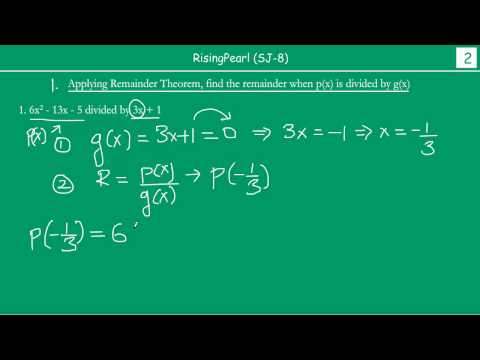Related tests